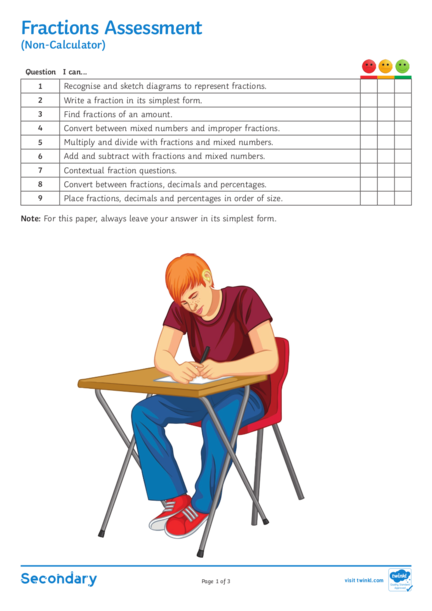# Fractions Assessment (non-calculator)Contributed by:Fractions Assessment year 4
1. Fractions Assessment
Question I can...
1 Recognise and sketch diagrams to represent fractions.
2 Write a fraction in its simplest form.
3 Find fractions of an amount.
4 Convert between mixed numbers and improper fractions.
5 Multiply and divide with fractions and mixed numbers.
6 Add and subtract with fractions and mixed numbers.
7 Contextual fraction questions.
8 Convert between fractions, decimals and percentages.
9 Place fractions, decimals and percentages in order of size.
Note: For this paper, always leave your answer in its simplest form.
Page 1 of 3 visit twinkl.com
2. Fractions Assessment
(1)
b. Shade 3/5 of this shape:
(1)
25
a. Write 40 in its simplest form.
(1)
84
b. Write 108
in its simplest form.
(1)
a. Find 1/7 of £42.
(1)
b. Find 3/8 of £72.
(2)
c. Find 2/5 of £19.
(2)
27
a. Write 4 as a mixed number.
(1)
b. Write 54/9 as an improper fraction.
(1)
Page 2 of 3 visit twinkl.com
3. Fractions Assessment
a. Evaluate 4/9 ÷ 2/5
(2)
b. Evaluate 31/2 × 5/7
(2)
a. Evaluate 1/3 + 3/4
(3)
b. Evaluate 22/7 − 13/5
(3)
7. A pack of soil weighs 41/5 lbs. Each plant pot requires just 13/4 lbs of soil.
Calculate how many plant pots can be filled.
(4)
8. Complete the table:
Fractions Decimals Percentages
0.4
57%
3
20
2.9%
(4)
9. Write these numbers in order of size, starting with the smallest:
13
67%, 20 , 0.656
(2)
Page 3 of 3 visit twinkl.com
Book a Trial Class
Related Tutorials
Fun with Fractions